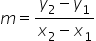Mathematics
Easy

Question

# Find the slope of the line that passes through (2, 4) and (6, 12)

## 1/2-1/22-2Hint:

## The correct answer is: 2

### Given Points (2, 4) and (6, 12).Step 1 : Slope of a line that passes through (x1,y1), (x2,y2 ) isstep 2 : substituting the values .= 12 - 4 / 6 - 2= 8 / 4=2 , is the slope of line, m is slope
and it passes through ( x1 , y1 ) and ( x2 , y2 ) .

### Related Questions to study#### With Turito Foundation.#### Get an Expert Advice From Turito.